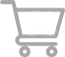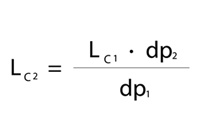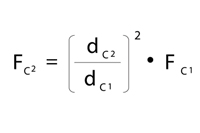EN0 Compare
Add products to the comparison using the scales icon and here you can then compare their parameters.0 Basket
Your basket is empty...# Scale down in UHPLC

Scale-down procedure from conventional HPLC to UHPLC requires optimizing columns selectivity and efficiency. As soon as we have finished this method development, we can perform a scale-down procedure. A few simple calculations can be used to determine equivalent run conditions. This article descibes them sequentially.

####Adjusting Column Size

The first calculation determines the appropriate column length. Keeping the same column length while decreasing the particle size will increase the number of theoretical plates in that given column length. Therefore, column length can be shortened without losing resolution. By using Equation 1 and when adjusting the column length properly, we can maintain the same separation.

#### Adjusting Injection Volume

Once we have determined the proper column length, we can determine the appropriate injection volume. Decreasing the column internal diameter and length, decreases the overall column volume and sample capacity. Therefore, we must alter the injection volume as described in Equation 2. Please note that since overall column volume has decreased, it is important to match the sample solvent to the starting mobile phase composition. Mismatched sample solvents can cause irreproducible retention times, efficiencies, and even changes in selectivity.

####Adjusting Flow Rate

Flow rate must be adjusted to maintain comparable linear velocity through a column with smaller internal diameter. Linear velocity is defined as the distance mobile phase travels over time, whereas flow rate is the volume of mobile phase that travels over time. To maintain the same linear velocity, which is important to maintain efficiencies, flow rates must be decreased as column internal diameter decreases. Also, since smaller particle sizes give rise to higher optimal linear velocities, isocratic flow rates should be calculated with particle size taken into account. Equation 3 can be used to simply and quickly estimate the adjusted flow rate needed for equivalent chromatography. It is also important to note that <2µm particle sizes are less affected by higher flow rates, and therefore faster flow rates can be used in isocratic systems without detrimental effects on peak efficiency.

#### Adjusting Time Program

Lastly, after we have determined the proper column length, injection volume, and flow rate, we can find the equivalent time needed for gradient or step elutions. As an analytical method is scaled down, the time program needs to also be scaled down to keep the phase interactions the same. Time can be adjusted using Equation 4.

Article referene: Rick Lake, Restek Coroporation